# I calculate in excel lead to logic blocks

hello i’m lost. I need the 11 numbers to be multiplied with the fixed numbers that algorithms call that are: 5432765432
I must multiply and then divide by 11 the result take an integer part and subtract -2 the result if it is greater than 0 I must subtract it minus 11 that result must be equal to the last number that I insert.

calc: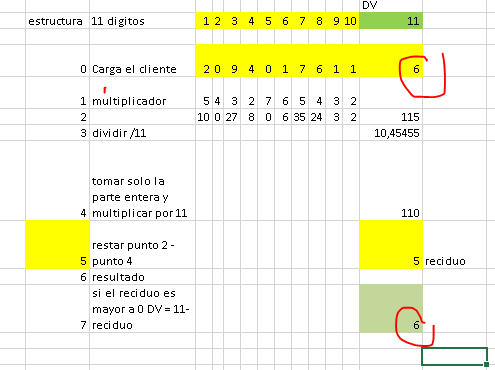block: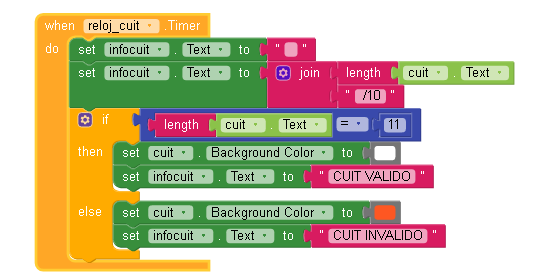separate them into lists
1 for numbers
1 for multipliers
1 for multiplication result

Then read this list item by item and add them up with an accumulator

http://www.imagnity.com/tutorials/app-inventor/list-blocks-on-app-inventor/

Thank you very much for your answer, a little guide me but I will read what you send me, to be able to understand it better.

1 Like

The number that comes from the customer comes all together, right?
11 digits together, right?
You , perhaps , need to take it one by one :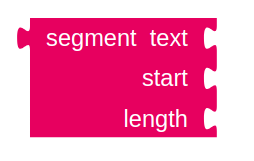And Maybe you wiil not need list…

If the user must enter it all together like this, but the last number I call it an identifier number because with that number doing the calculation it must return the same number in this case it is 6, if it does not give me 6 it is because the number that I entered was misspelled, because the calculation works for me in my excel.

screen:1 Like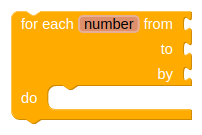Take this value (which is size 11)
Insert in the yellow block​, use the red blockStart ->number
Lenght ->1

aboveto take each digit and multiply by the respective multiplier…

From 1
To 11
By 1

of course I attach it there
1-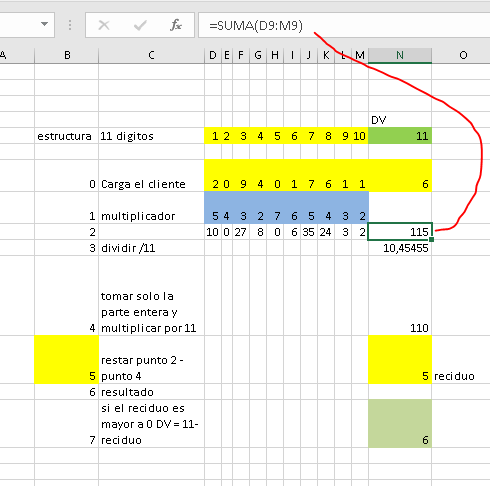2-3-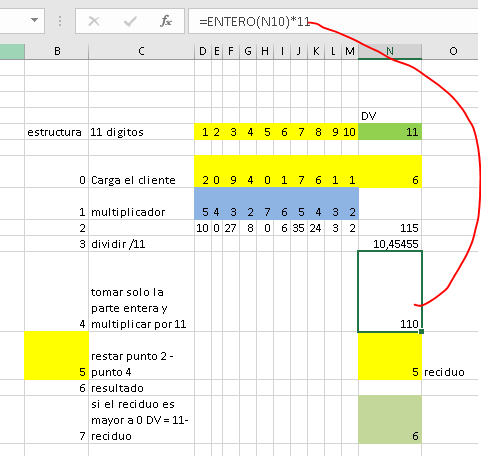4-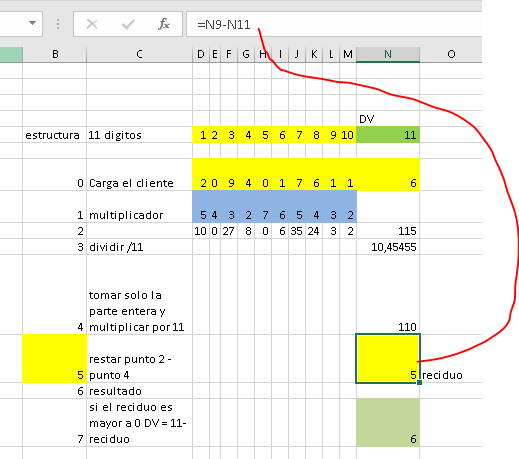5-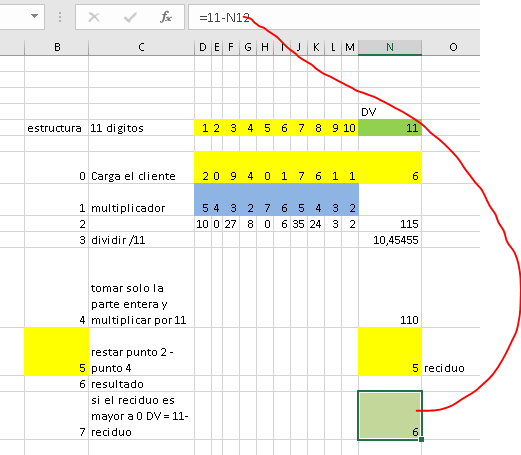1 Like

Multiply and accumulate inside yellow block

set total = total + (digit x multiplier)

Thank you very much for your kindness and help, orient me much better now, I appreciate.

1 Like

How do you optain first 6 ?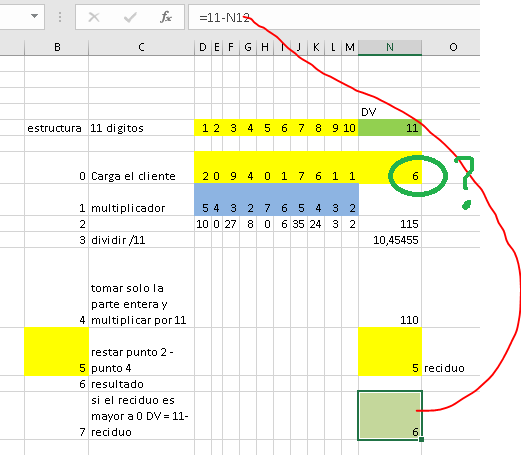It is part of the number to be entered

It is usually the last digit of your number that was generated by the algorithm.
It’s digit verifier
It is part of each customer’s number (in This case , digit 11)

So with the first 10 digits of the example, the last will always be 6. If you enter another number, it will not be valid.

#off-topic Now it’s time to go to courtSo actually you want to check true or false, valid or not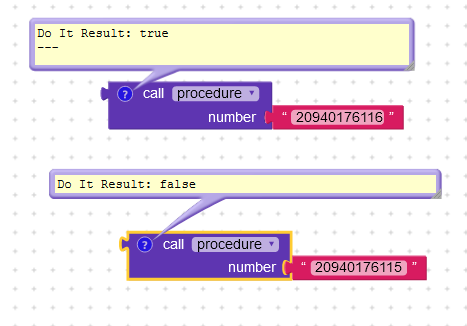Modified procedure to check if contains letters or if number doesn’t have 11 digits (I used regex extension by @Kevinkun )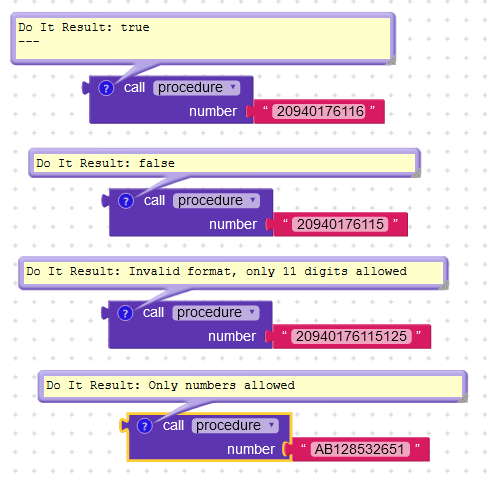1 Like

Excuse me for the late answer, I had no connection, the blocks that it shows me are based on the example excel that I published? because I would appreciate it very much

Yes since I believe seeing your excel example that you want to check whether an Argentinian VAT number is valid or not.

correct, where the user can put any cuit with only 11 digits and the excel calculation what verifies is that what input is valid or not.

.Yes , taking the multiplier number (5432765432) and putting it on Google , we found this information 🤷

They are “routines” or “algorithms” to generate a number with your own verification digit (it exists worldwide - credit cards, bank accounts, social security number)

1 Like

Try this aia

argentinian_vat.aia (12.1 KB)